Question 1

If the dimensions of a physical quantity are given by [MaLbTc], then the physical quantity will be :

A : Force if a = 0, b = -1, c = -2
B : Pressure if a = 1, b = -1, c = -2
C : Velocity if a = 1, b = 0, c = -1
D : Acceleration if a = 1, b = 1, c = -2

•
.

Solution :
[P] = F/A = [MLT-2/L2] = [ML-1T-2]

•   Question 2

A particle starts its motion from rest under the action of a constant force. If the distance covered in first 10 seconds is S1 and that covered in the first 20 seconds is S2 then :

A : S2 = S1
B : S2 = 2S1
C : S2 = 3S1
D : S2 = 4S1

•
.

Solution :
s =1\2 * at2

•   Question 3

A bus is moving with a speed of 10ms-1 on a straight road. A scooterist wishes to overtake the bus in 100s. If the bus is at a distance of 1 km from the scooterist, with what speed should the scooterist chase the bus ?

A : 10ms-1
B : 20ms-1
C : 40ms-1
D : 25ms-1

•
.

Solution :
Sr = vrt 1000 = (v - 10) * 100 v = 20 m/s

•   Question 4

The mass of lift is 2000 kg. When the tension in the supporting cable is 28000 N, then its acceleration is :

A : 14 ms-2 upwards
B : 30 ms-2 downwards
C : 4 ms-2 upwards
D : 4 ms-2 downwards

•
.

Solution :
F = ma
T - mg = ma
a =(T- mg)/m
=4 m/s2

•   Question 5

An explosion blows a rock into three parts Two parts go off at right angles to each other. These two are, 1 kg first part moving with a velocity of 12 ms-1 and 2 kg second part moving with a velocity of 8 ms-1. If the thirds part files off with a velocity of 4 ms-1, its mass would be :

A : 3kg
B : 5kg
C : 7kg
D : 17kg

•
.

Solution :
P̃1 + P̃2 + P̃3 = 0
|P̃3| =| P̃1 + P̃2| m * 4 = √(P12 + P22)

•   Question 6

A block of mass M is attached to the lower end of a vertical spring. The spring is hung from a ceiling and has force constant value k. The mass is released from rest with the spring initially unstretched. The maximum extension produced in the length of the spring will be

A : Mg/2k
B : Mg/k
C : 2 Mg/k
D : 4 Mg/k

•
.

Solution :
Loss in grav. PE = gain in spring PE At maximum elongation
Mgx =1/2 * kx2
x = (2Mg)/k

•   Question 7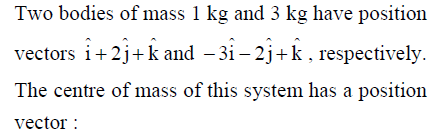A :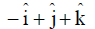B :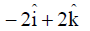C :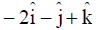D :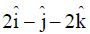•
.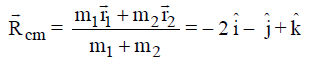•   Question 8

Four identical thin rods each of mass M and length l, from a square frame. Moment of inertia of this frame about an axis through the centre of the square and perpendicular to its plane is :

A : 1/3 Ml2
B : 4/3 Ml2
C : 2/3 Ml2
D : 13/3 Ml2

•
.

Solution :
I = 4 * [(Ml2)/12 + M(l/2)2] the parallel axis theorem =
4/3 Ml2

•   Question 9

A thin circular ring of mass M and radius R is rotating in a horizontal plane about an axis vertical to its plane with a constant angular velocity w, If two objects each mass m be attached gently to the opposite ends of a diameter of the ring, the ring, will then rotate with an angular velocity :

A : wM/(M+m)
B : w(M-2m)/(M+2m)
C : w(M)/(M+2m)
D : w(M + 2m)/(M)

•
.

Solution :
Apply conservation of angular momentum
Li= Lf
MR2w = (M + 2m)R2w'
w' = Mw/(M+2m)

•   Question 10

A body, under the action of a force F̃ = 6 î - 8 ĵ +10 k̂, acquires an acceleration of 1 m/s2. The mass of this body must be :

A : 10 √2 kg
B : 2 √10 kg
C : 10 kg
D : 20 kg

•
.

Solution :
F̃ = 6 î - 8 ĵ +10 k̂
|F|= √(62 + 82 +102)
= 10 √2
m= |F|/1 = 10 √2 kg

•   Question 11

If F̃ is the force acting on a particle having position vector r̃ and t̃ be the torque of this force about the origin, then :

A : r̃. t̃ = 0 and F̃. t̃ != 0
B : r̃. t̃ != 0 and F̃. t̃ = 0
C : r̃. t̃ > 0 and F̃. t̃ < 0
D : r̃ . t̃ = 0 and F̃. t̃ = 0

•
.

Solution :
t̃ is perpendicular to r̃ and F̃

•   Question 12

The figure shows elliptical orbit of a planet m about the sun S. The shaded area SCD is twice the shaded are SAB. It t1 is the time for the planet to move from C to D and t2 is the time to move from A to B then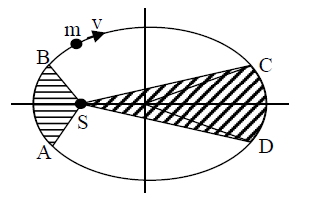A : t1 = t2
B : t1 > t2
C : t1 = 4t2
D : t1 = 2t2

•
.

Solution :
Kepler's 2nd law
A1 / t1 = A2 / t2
2A /t1 = A /t2

•   Question 13

An engine pumps water continuously through a hose. Water leaves the hose with a velocity v and m is the mass per unit length of the water jet. What is the rate which kinetic energy is imparted to water ?

A : 1/2 m2v2
B : 1/2 mv 3
C : mv3
D : 1/2 mv2

•
.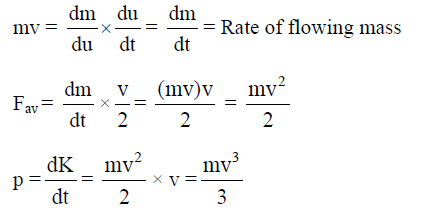•   Question 14

A body of mass 1 kg of thrown upwards with a velocity 20 m/s. It momenetarily comes to rest after attaining a height of 18m. How much energy is lost due to air friction ? (g = 10 m/s2)

A : 10 J
B : 20 J
C : 30 J
D : 40 J

•
.

Solution :
Loss of energy =1/2 mv2 - mgh = 20 J

•   Question 15

The two ends of a rod of length L and a uniform cross-sectional area A are kept at two temperatures T1 and T2 (T1 > T2). The rate of heat transfer,dt/dQ , through the rod in a steady state is given by :

A : dQ/dt =kA(T1 - T2 )/ L
B : dQ/dt =kL(T1 - T2 )/ A
C : dQ/dt =k(T1 - T2 )/ LA
D : dQ/dt =kLA(T1 - T2 )

•
.

Solution :
For steady state dQ/dt = kA(T1-T2)/L

•   Question 16

In thermodynamic processes which of the following statements is not true ?

A : In an adiabatic process PV γ = constant
B : In an adiabatic process the system is insulated from the surroundings
C : In an isochoric process pressure remains constant
D : In an isothermal process the temperature remains constant

•
.

Solution :
Isochoric -> Volume constant

•   Question 17

A black body at 227°C radiates heat at the rate of 7 cals/cm2s. At a temperature of 727°C, the rate of heat radiated in the same units will be :

A : 80
B : 60
C : 50
D : 112

•
.

Solution :
P directly proportional to T4
P2 / P1 = (1000 /500)4

•   Question 18

The internal energy change in a system that has absorbed 2 k cal of heat and done 500 J of work is :

A : 7900 J
B : 8900 J
C : 6400 J
D : 5400 J

•
.

Solution :
dU = Q - W = 8400 - 500 = 7900 J

•   Question 19

The driver of a car traveling with speed 30 m/sec towards a hill sounds a horn of frequency 600 Hz. If the velocity of sound in air is 330 m/s, the frequency of reflected sound as heard by driver is :

A : 500 Hz
B : 550 Hz
C : 555.5 Hz
D : 720 Hz

•
.

Solution :
n'=v((c+u)/(c-u))
600(360/300) = 720

•   Question 20

A simple pendulum performs simple harmonic motion about x = 0 with an amplitude a and time period T. The speed of the pendulum at x = a/2 will be :

A : πa√3 / T
B : πa√3 / 2T
C : πa / T
D : 2a / T

•
.

Solution :
v = w √(A2 ? x2)
2π/T * √(a2 - a2/4)
(πa√3)/T

•   Question 21

Which one of the following equations of motion represents simple harmonic motion ?
Where k, k0, k1 and a are all positive

A : Acceleration = kx
B : Acceleration = - k0x + k1x2
C : Acceleration = - k (x + a)
D : Acceleration = k(x + a)

•
.

Solution :
In SHM, Frestoring ∝ -x

•   Question 22

The electric field part of an electromagnetic wave in a medium is represented by : Ex = 0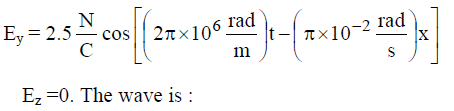A : Moving along -x direction with frequency 106 Hz and wavelength 200m
B : Moving along y direction with frequency 2π*106 Hz and wavelength 200m
C : Moving along x direction with frequency 106 Hz and wavelength 100m
D : Moving along x direction with frequency 106 Hz and wavelength 200m

•
.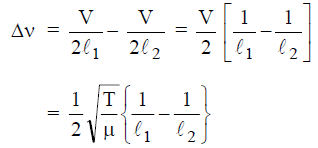•   Question 23

A wave in a string has an amplitude of 2 cm. The wave travels in the +ve direction of x-axis with a speed of 128 m/s and it is noted that 5 complete waves fit in 4 m length of the string. The equation describing the wave is

A : y = (0.02)m sin (7.58x - 1005 t)
B : y = (0.02)m sin (7.85x + 1005 t)
C : y = (0.02)m sin (15.7x - 2010 t)
D : y = (0.02)m sin (15.7x + 2010 t)

•
.

Solution :
5 λ = 4
λ = 4/5
k =2 π/ λ
= 7.85
wave moves along positive X-direction

•   Question 24

Each of the two strings of length 51.6 cm and 49.1 cm are tensioned separately by 20 N force. Mass per unit length of both the strings is same and equal to 1 g/m. When both the strings vibrate simultaneously the number of beats is :

A : 3
B : 5
C : 7
D : 8

•
.•   Question 25

Three capacitors each of capacitance C and of breakdown voltage V are joined in series. The capacitance and breakdown voltage of the combination will be :

A : 3C, 3V
B : C/3 , V/3
C : 3C , V/3
D : C/3 ,3V

•
.

Solution :
In series, Ceq = C/3
Veq=3V

•   Question 26

A wire of resistance 12 ohms per metre is bent to form a complete circle of radius 10 cm. The resistance between its two diametrically opposite points, A and B as shown in the figure, is :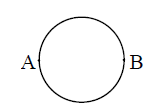A : 6 ohm
B : 0.6πohm
C : 3 ohm
D : 6πohm

•
.

Solution :
Total resistance of wire = 12 ohm * 2π * 10-1
= 2.4π
Resistance of each half = 1.2π and as about diameter both parts are in parallel Req. = 1.2π/2 = 0.6π ohm

•   Question 27

A bar magnet having a magnetic movement of 2 * 104 JT-1 is free to rotate in a horizontal plane. A horizontal magnetic field B = 6 * 10�4 T exists in the space. The work done in taking the magnet slowly from a direction parallel to the field to a direction 60° from the field is :

A : 2J
B : 0.6J
C : 12J
D : 6J

•
.

Solution :
W = MB(cosΘ1 - cosΘ2)
2 * 104 * 6 * 10-4 (cosΘ - cos60)
=12 * 1/2 = 6 J

•   Question 28

The magnetic force acting on a charged particle of charge -2µc in a magnetic field of 2T acting in y direction, when the particle velocity is (2î + 3ĵ) * 106 ms-1, is :

A : 8N in z - direction
B : 8N in z - direction
C : 4N in z - direction
D : 8N in y - direction

•
.

Solution :
F̃ = q(Ṽ * B̃)
- 2 * 10-6(2î + 3ĵ) * 106 * 2ĵ]
(-8N)k̂

•   Question 29

A conducting circular loop is placed in a uniform magnetic field 0.04T with its plane perpendicular to the magnetic field. The radius of the loop starts shrinking at 2 mm/s. The induced emf in the loop when the radius is 2 cm is :

A : 1.6 πµv
B : 3.2 πµv
C : 4.8 πµv
D : 0.8 πµv

•
.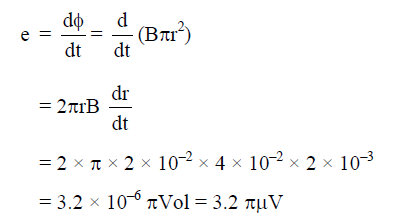•   Question 30

The electric potential at a point (x, y, z) is given by V = - x2y - xz3 + 4 The electric field Ẽ at that point is :

A : Ẽ = î(2xy - z3) + ĵxy2 + k̂3z2x
B : Ẽ = î(2xy + z3) + ĵx2 + k̂3xz2
C : Ẽ = î(2xy) + ĵ(x2 + y2 )+ k̂(3xz-y2)
D : Ẽ = îz + ĵxyz + k̂z2

•
.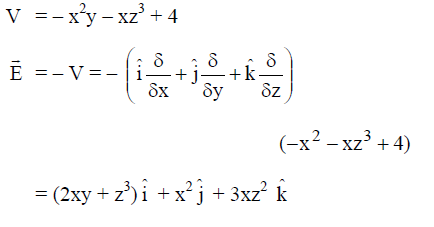•   Question 31

See the electrical circuit shown in this figure. Which of the following equations is a correct equation for it ?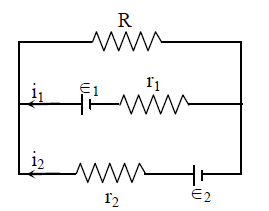A : ξ1 - (i1 + i2)R + i1r1 = 0
B : ξ1 - (i1 + i2)R - i1r1 = 0
C : ξ2 - (i1r2) - ξ1 - i1r1 = 0
D : 2 - (i1 + i2)R + i2r2 = 0

•
.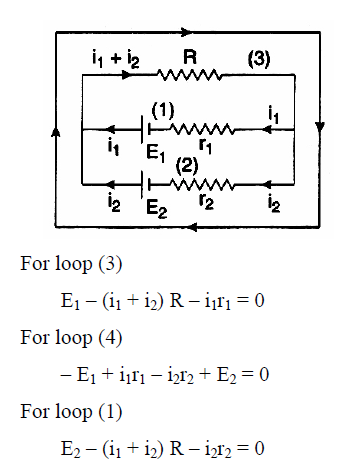•   Question 32

A galvanometer having a coil resistance of 60 ohm shows full scale deflection when a current of 1.0 amp passes through it. It can be converted into an ammeter to read currents upto 5.0 amp by :

A : Putting in parallel a resistance of 15 ohm
B : Putting in parallel a resistance of 240 ohm
C : Putting in series a resistance of 15 ohm
D : Putting in series a resistance of 240 ohm

•
.

Solution :
Ig = 1.0A, G = 60q, I = 5.0 A
S = Ig/ (I -Ig )* G
S = 1.0/ (5.0 -1.0)*60
=15 ohm in parallel

•   Question 33

Under the influence of a uniform magnetic field, a charged particle moves with constant speed V in a circle of radius R. The time period of rotation of the particle :

A : Depends on both v and R
B : Depends on v and not on R
C : Depends on R and not on v
D : Is independent of both v and R

•
.

Solution :
T = 2πm/2B

•   Question 34

Power dissipated in an LCR series circuit connected to an a.c. source of emf ξ is :

A :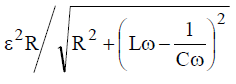B :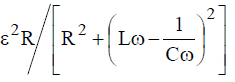C :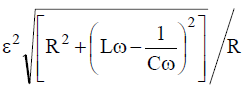D :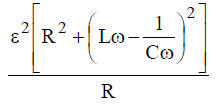•
.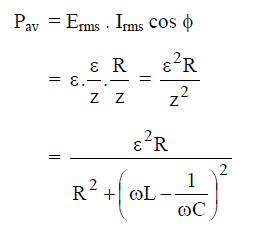•   Question 35

Three concentric spherical shells have radii a, b, and c(a < b < c) and have surface charge densities σ , -σ and σ respectively. If VA, VB and VC denote the potentials of the three shells, then, for c = a + b, we have :

A : VC = VB = VA
B : VC = VA != VB
C : VC = VB != VA
D : VC != VB != VA

•
.

Solution :
qA = 4πa2σ,
qB = - 4πb2σ,
qC = 4πc2σ,
c = a + b
VA = 1/(4πε0) * (qA/a + qB/b + qC/c)
VA = 2σa / ε0
Similarly VB = 2σ/ ε0 * (a + a2/b) and VC = 2σa / ε0
So VC = VA != VB

•   Question 36

A student measures the terminal potential difference (V) of a cell (of emf ξ and internal) resistance r) as a function of the current (I) flowing through it. The slope and intercept of the graph between V and I, then respectively equal to :

A : - ξ and r
B : ξ and -r
C : - r and ξ
D : r and -ξ

•
.

Solution :
E = V + Ir
V = E - Ir
Comparing with y = mx + c
Slope = - r, intercept = E

•   Question 37

A rectangular, a square, a circular and an elliptical loop, all in the (x - y) plane, are moving out of a uniform magnetic field with a constant velocity,Ṽ = v.î .The magnetic field is directed along the negative z-axis direction. The induced emf, during the passage of these loops, come out of the field region, will not remain constant for :

A : any of the four loops
B : The rectangular, circular and elliptical loops
C : The circular and the elliptical loops
D : Only the elliptical loop

•
.

Solution :
Out of the four structures, when the circular and elliptical loops come out from the field, equal are is not traced in equal interval of time. So any induced in both is not constant.

•   Question 38

If a diamagnetic substance is brought near the north or the south pole of a bar magnet, it is :

A : Attracted by both the poles
B : Repelled by both the poles
C : Repelled by the north pole and attracted by the south pole
D : Attracted by the north pole and repelled by the south pole

•
.

Solution :
As diamagnetic substances have negative intensity of magnetisation, they are weekly repelled by the external field.

•   Question 39

The number of photoelectrons emitted for light of a frequency v (higher than the threshold frequency v0) is proportional to :

A : Frequency of light (v)
B : v - v0
C : Threshold frequency (v0)
D : Intensity of light

•
.

Solution :
No. of photoelectrons emitted is independent of frequency but depends on intensity.

•   Question 40

Monochromatic light of wavelength 667 nm is produced by a helium neon laser. The power emitted is 9 mW. The number of photons arriving per second on the average at a target irradiated by this beam is :

A : 3 * 1019
B : 9 * 1017
C : 3 * 1016
D : 9 * 1015

•
.

Solution :
No. of photons = E/(hc/λ)

•   Question 41

The figure shows a plot of photo current versus anode potential for a photo sensitive surface for three different radiations. Which one of the following is a correct statement ?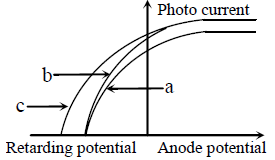A : Curves (b) and (c) represent incident radiations same frequencies having same intensity.
B : Curves (a) and (b) represent incident radiations of different frequencies and different intensities
C : Curves (a) and (b) represent incident radiations of same frequencies but of different intensities
D : Curves (b) and (c) represent incident radiations of different frequencies and different intensities

•
.

Solution :
As a and b have same stopping potential and c has greater stopping potential, then vc > va = vb as b and c have same saturation current and a has lesser value.

•   Question 42

The number of beta particles emitted by a radioactive substance is twice the number of alpha particles emitted by it. The resulting daughter is an :

A : Isotope of parent
B : Isobar of parent
C : Isomer of parent
D : Isotone of parent

•
.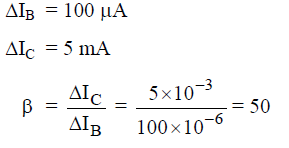•   Question 43

The ionization energy of the electron in the hydrogen atom in its grounds state is 13.6 eV. The atoms are excited to higher energy levels to emit radiations of 6 wavelengths. Maximum wavelength of emitted radiation corresponds to the transition between :

A : n = 4 to n = 3 states
B : n = 3 to n = 2 states
C : n = 3 to n = 1 states
D : n = 2 to n = 1 states

•
.

Solution :
n(n-1)/2 = 6
n = 4
For maximum wavelength energy difference between states should be minimum because
λ = hc/delE

•   Question 44

In a Rutherford scattering experiment when a projectile of charge Z1 and mass M1 approaches a target nucleus of charge Z2 and mass M2, the distance of closest approach is r0. The energy of the projectile is :

A : Directly proportional to mass M1
B : Directly proportional to M1 * M2
C : Directly proportional to Z1Z2
D : Inversely proportional to Z1

•
.

Solution :
Energy = 1/4πε0 * Z1Z2/r0

•   Question 45

In the nuclear decay given below :
The particles emitted in the sequence are :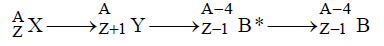A : α , β , γ
B : β , α , γ
C : γ , β , α
D : β , γ ,α

•
.•   Question 46

The mean free path of electrons in a metal is 4 * 10-8 m. The electric field which can give on an average 2 eV energy to an electron in the metal will be in units of V/m :

A : 5 * 107
B : 8 * 107
C : 5 * 10-11
D : 8 * 10-11

•
.

Solution :
qV = 2eV
1.6 * 10-19V = 2 * 1.6 * 10-19 V
E = V/d
E = 2V/4* 10 -8
= 5 * 107

•   Question 47

Sodium has body centred packing. Distance between two nearest atoms is 3.7 Å. The lattice parameter is :

A : 8.6 Å
B : 6.8 Å
C : 4.3 Å
D : 3.0 Å

•
.

Solution :
√3 a/2 = 3.7 Å
a = 2*3.7/√3 = 4.3 Å

•   Question 48

A p-n photodiode is fabricated from a semiconductor with a band gap of 2.5 eV. It can detect a signal of wavelength

A : 4960 Å
B : 6000 Å
C : 4000 nm
D : 6000 nm

•
.

Solution :
λmax =hc/eV
4960 Å

•   Question 49

The symbolic representation of four logic gates are given below :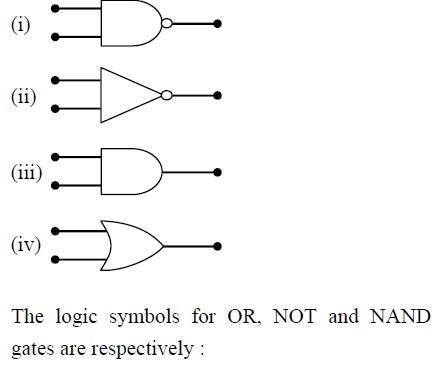A : (i), (iii), (iv)
B : (iii), (iv), (ii)
C : (iv), (i), (iii)
D : (iv), (ii), (i)

•
.•   Question 50

A transistor is operated in common-emitter configuration at VC = 2V such that a change in the base current from 100µA to 200µA produces a change in the collector current from 5 mA to 10 mA. The current gain is :

A : 50
B : 75
C : 100
D : 150

•
.•   Question 51

10 g of hydrogen and 64 g of oxygen were filled in a steel vessel and exploded. Amount of water produced in this reaction will be

A : 1 mol
B : 2 mol
C : 3 mol
D : 4 mol

•
.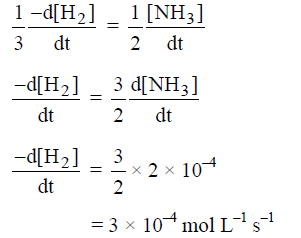•   Question 52

Oxidation number of P in PO3-4 , of S in SO2-4 and that of Cr in Cr2O2-7 are respectively

A : 3, 6 and 6
B : 5, 6 and 6
C : 3, 6 and 5
D : 5,3 and 6

•
.

•   Question 53

Maximum number of electrons in a subshell or an atom is determined by the following :

A : 2n2
B : 4l + 2
C : 2l + 2
D : 4l - 2

•
.

Solution :
Maximum no. of electrons in any subshell = 4l + 2

•   Question 54

Which of the following is not permissible arrangement of electrons in an atom ?

A : n = 3, l = 2, m = -3, s = -1/2
B : n = 4, l = 0, m = 0, s =-1/2
C : n = 5, l = 3, m = 0, s = 1/2
D : n = 3, l = 2, m = -3, s=-1/2

•
.

Solution :
m value - l to + l

•   Question 55

From the following bond energies : H - H bond energy : 431.37 kJ mol-1 C = C bond energy : 606.10 kJ mol-1 C - C bond energy : 336.49 kJ mol-1 C - H bond energy : 410.50 kJ mol-1 Enthalpy for the reaction,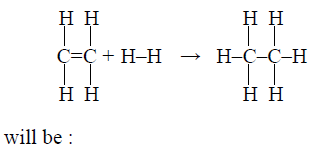A : 553.0 kJ mol-1
B : 1523.6 kJ mol-1
C : -243.6 kJ mol-1
D : -120.0 kJ mol-1

•
.

Solution :
ΔH = dissociation energy of reactant - Bond dissociation of energy of product.
ΔH = (606.10 + 4 * 410.5 + 431.37)- (6 * 410.50 + 336.49)
= - 120.0 kJ/mol

•   Question 56

The ionization constant of ammonium hydroxide is 1.77 * 10-5 at 298 K. Hydrolysis constant of ammonium chloride

A : 5.65 * 10-12
B : 5.65 * 10-10
C : 6.50 * 10-12
D : 5.65 * 10-13

•
.

Solution :
Kh = Kw / Kb = 5.65 * 10�10

•   Question 57

Given :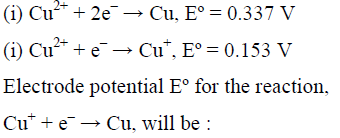A : 0.38 V
B : 0.52 V
C : 0.90 V
D : 0.30 V

•
.•   Question 58

What is the [OH-] in the final solution prepared by mixing 20.0 mL of 0.050 M HCl with 30.0 mL of 0.10 M Ba(OH)2 ?

A : 0.12 M
B : 0.10 M
C : 0.40 M
D : 0.0050 M

•
.

Solution :
20 mL of 0.50 M HCl = 20 * 0.050 m mol = 1.0 m mol = 1.0 meq. of HCl
30 mL of 0.10 M Ba(OH)2
= 30 * 0.1 m mol
= 3m mol = 3 * 2 meq
= 6 meq Ba(OH)2
1 meq of HCl will neutralize 1 meq of Ba(OH)2
Ba(OH)2 left = 5 meq.
Total volume = 50 mL
Ba(OH)2 conc. in final solution = 5/50N = 0.1N = 0.05M
[OH-] = 2 * 0.05 M = 0.10 M

•   Question 59

The energy absorbed by each molecule (A2) of a substance is 4.4 * 10-19 J and bond energy per molecule is 4.0 * 10-19 J. The kinetic energy of the molecule per atom will be :

A : 4.0 * 10-20 J
B : 2.0 * 10-20 J
C : 2.2 * 10-19 J
D : 4 * 10-19 J

•
.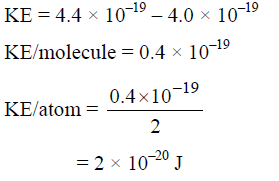•   Question 60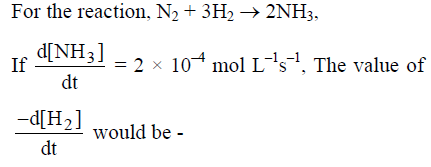A : 1 * 10-4 mol L-1 s-1
B : 3 * 10-4 mol L-1 s-1
C : 4 * 10-4 mol L-1 s-1
D : 6 * 10-4 mol L-1 s-1

•
.•   Question 61

For the reaction A + B -> products, it is observed that :
(1) On doubling the initial concentration of A only, the rate of reaction is also doubled and
(2) On doubling the initial concentration of both A and B, there is a change by a factor of 8 in the rate of the reaction. The rate of this reaction is given by :

A : rate = k[A] [B]
B : rate = k[A]2[B]
C : rate = k[A][B]2
D : rate = k[A]2[B]2

•
.

Solution :
Rate = k[A][B]2
= k[2A][2B]2
= k * 8[A][B]2

•   Question 62

The equivalent conductance of M/32 solution of a weak monobasic acid is 8.0 mho cm2 and at infinite dilution is 400 mho cm2, The dissociation constant of this acid is

A : 1.25 * 10-4
B : 1.25 * 10-5
C : 1.25 * 10-6
D : 6.25 * 10-4

•
.

Solution :
α = AC / A
=8/400
Ka = C α2
=1/32 * 8/400 * 8/400
1.25 * 10-5

•   Question 63

A 0.0020 M aqueous solution of an ionic compound Co(NH3)5(NO2)Cl freezes at - 0.00732°C. Number of moles of ions which 1 mole of ionic compound produces on being dissolved in water will be : (kf = 1.86°C/m)

A : 1
B : 2
C : 3
D : 4

•
.

Solution :
Use ΔTf = ikf . m

•   Question 64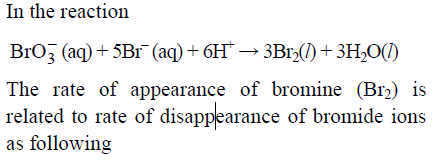A :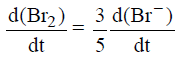B :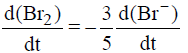C :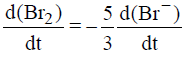D :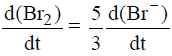•
.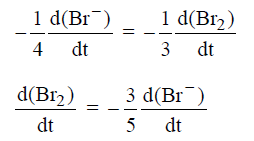•   Question 65

Lithium metal crystallizes in a body centred cubic crystal. If the length of the side of the unit cell of lithium is 351 pm, the atomic radius of lithium will be :

A : 300.5 pm
B : 240.8 pm
C : 151.8 pm
D : 75.5 pm

•
.

Solution :
for bcc type of unit cell
(√3a)/4 = r
Put the values
=151.98

•   Question 66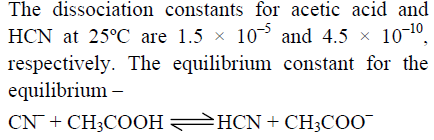A : 3.0 * 104
B : 3.0 * 105
C : 3.0 * 10-5
D : 3.0 * 10-4

•
.

Solution :
Kc = Ka(CH3COOH) * 1/Ka(HCN)
=1.5 * 10-5 * 1/(4.5 * 10-10)

•   Question 67

The values of ΔH and ΔS for the reaction, C(graphite) + CO2(g) -> 2CO(g) are 170 kJ and 170 JK-1 respectively. This reaction will be spontaneous at

A : 510 K
B : 710 K
C : 910 K
D : 1110 K

•
.

Solution :
For a spontaneous reaction
ΔG = -ve
Or ar eq. ΔG = 0
ΔH = TΔS
T = ΔH /ΔS
170* 1000/170 = 1000K

•   Question 68

Half-life period of a first-order reaction is 1386 seconds. The specific rate constant of the reaction is :

A : 5.0 * 10-2 s-1
B : 5.0 * 10-3 s-1
C : 0.5 * 10-2 s-1
D : 0.5 * 10-3 s-1

•
.

Solution :
K = 0.693/ t1/2= 0.693/1386 =0.5 * 10-3 s-1

•   Question 69

In which of the following molecules / ions BF3,NO2- ,NH2- ,H2O the central atom is sp2

A : BF3 and NO2-
B : NO2- and NH2-
C : NH2- and H2O
D : NO2- and H2O

•
.

Solution :
Both BF3 and NO2- are sp2 hybridized.

•   Question 70

Among the following which is the strongest oxidizing agent ?

A : Cl2
B : F2
C : Br2
D : I2

•
.

Solution :
F2 -> reduction potential very high so strongest oxidizing agent.

•   Question 71

According to MO theory which of the following lists ranks the nitrogen species in terms of increasing bond order :

A :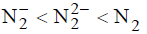B :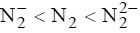C :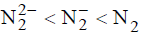D :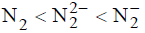•
.

Solution :
N2 = 14e = B.O. = 3
N2- = 15e = B.O. = 2.5 N22- = 16e = B.O.= 2

•   Question 72

In the case of alkali metals, the covalent character decreases in the order :

A : MI > MBr > MCl > MF
B : MCl > MI > MBr > MF
C : MF > MCl > MBr > MI
D : MF > MCl > MI > MBr

•
.

Solution :
MI > MBr > MCl > MF Down the group increases covalent character

•   Question 73

Which of the following oxides is not expected to react with sodium hydroxide ?

A : BeO
B : B2O3
C : CaO
D : SiO2

•
.

Solution :
Since CaO itself is basic, It will not react with NaOH

•   Question 74

Al2O3 is reduced by electrolysis at low potentials and high currents. If 4.0 * 104 amperes of current is passed through molten Al2O3 for 6 hours, what mass of aluminium is produced ? (Assume 100% current efficiency, at. mass of Al = 27 g mol-1)

A : 1.3 * 104 g
B : 9.0 * 103 g
C : 8.1 * 104 g
D : 2.4 * 105 g

•
.

Solution :
Solve W = E/96500 * I * t

•   Question 75

The stability of +1 oxidation state increases in the sequence :

A : Ga < In < Al < Tl
B : Al < Ga < In < Tl
C : Tl < In < Ga < Al
D : In < Tl < Ga < Al

•
.

Solution :
+I stability down the group increase due to inert pair effect
Al < Ga < In < Tl

•   Question 76

Copper crystallizes in a face-centred cubic lattice with a unit cell length of 361 pm. What is the radius of copper atom in pm ?

A : 108
B : 128
C : 157
D : 181

•
.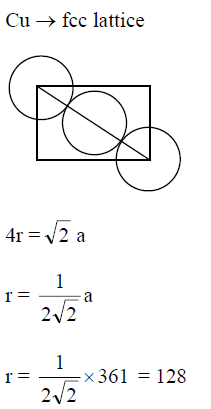•   Question 77

What is the dominant intermolecular force or bond that must be overcome in converting liquid CH3OH to a gas

A : London dispersion force
B : Hydrogen bonding
C : Dipole-dipole interaction
D : Covalent bonds

•
.

Solution :
Inter molecular force in alcohol is mainly H-bonding

•   Question 78

Which of the following complex ions is expected to absorb visible light ?
(At no. Zn = 30, Sc = 21, Ti = 22, Cr = 24)

A :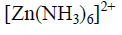B :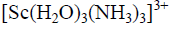C :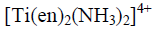D :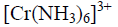•
.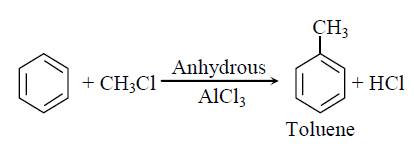•   Question 79

Out of Tif62- , CoF63- ,Cu2Cl2 and NiCl42- (Z of Ti = 22, Co = 27, Cu = 29, Ni = 28) the colourless species are :

A : CoF63- & NiCl42-
B : Tif62- & CoF63-
C : Cu2Cl2 & NiCl42-
D : Tif62- & Cu2Cl2

•
.

Solution :
In both Tif62- & Cu2Cl2, these no delectrons or no unpaired electrons so, these are colourless.

•   Question 80

Which of the following does not show optical isomerism
(en = Ethylenediamine)

A : [Co(en)3]3+
B : [Co(en)2Cl2]+
C : [Co(NH3)3Cl3]0
D : [Co(en)Cl2(NH3)2]+

•
.

Solution :
As complexes of the type [MA3B3] can show geometrical isomerism knows as facmer isomerism and not optical isomerism. So here [Co(NH3)3Cl3]0 can not show optical isomerism.

•   Question 81

Which one of the elements with the following outer orbital configurations may exhibit the largest number of oxidation states ?

A : 3d24s2
B : 3d34s2
C : 3d54s1
D : 3d54s2

•
.

Solution :
7th group -> largest number of oxidation state.

•   Question 82

Which of the following molecules acts as a Lewis acid ?

A : (CH3)3N
B : (CH3)3B
C : (CH3)2O
D : (CH3)3P

•
.

Solution :
(CH3)3B ->is electron efficient compound, so behaves as Lewis acid.

•   Question 83

Amongst the element with following electronic configurations, which one of them may have the highest ionization energy ?

A : [Ne]3s23p1
B : [Ne]3s23p3
C : [Ne]3s23p2
D : [Ar]3d104s2 4p3

•
.

Solution :
[Ne]3s23p3 ->has highest ionization energy (half-filled)

•   Question 84

The straight chain polymer is formed by

A : hydrolysis of (CH3)2SiCl2 followed by condensation polymerization
B : hydrolysis of (CH3)3SiCl followed by condensation polymerization
C : hydrolysis of CH3SiCl3 followed by condensation polymerization
D : hydrolysis of (CH3)4Si by addition polymerization

•
.•   Question 85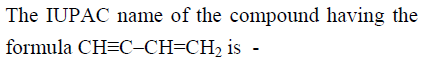A : 1-butene-3-yne
B : 3-buten-1-yne
C : 1-butyn-3-ene
D : but-1-yn-3-ene

•
.•   Question 86

Which of the following compounds will exhibit cis-trans (geometrical) isomerism ?

A : 1-Butanol
B : 2-Butene
C : 2-Butanol
D : 2-Butyne

•
.•   Question 87

H2COH.CH2OH on heating with periodic acid gives :

A :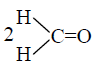B : 2CO2
C : 2HCOOH
D :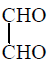•
.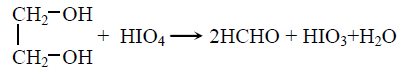•   Question 88

Consider the following reaction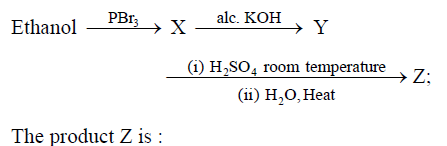A : CH3CH2-OH
B : CH2 = CH2
C : CH3CH2-O-CH2CH3
D : CH3CH2-O-SO3H

•
.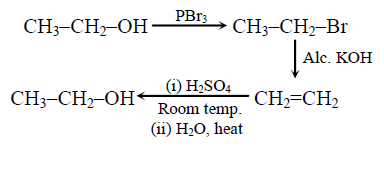•   Question 89

Benzene reacts with CH3Cl in the presence of anhydrous AlCl3 to form

A : Xylene
B : Toluene
C : Chlorobenzene
D : Benzylchloride

•
.•   Question 90

Nitrobenzene can be prepared from benzene by using a mixture of conc. HNO3 and conc. H2SO4. In the mixture, nitric acid acts as a/an

A : catalyst
B : reducing agent
C : acid
D : base

•
.

Solution :
HNO3 on nitrating mixture acts as a base.

•   Question 91

Which of the following reactions is an example of nucleophilic substitution reaction ?

A : RX + Mg -> RMgX
B : RX + KOH -> ROH + KX
C : 2RX + 2Na -> R-R + 2NaX
D : RX + H2 -> RH + HX

•
.

Solution :
RX + KOH -> ROH + KX
X- replaced by OH- show nucleophilic substitution reaction

•   Question 92

Which one of following is employed as a tranquilizer ?

A : Chlorpheninamine
B : Equanil
C : Naproxen
D : Tetracycline

•
.

•   Question 93

Structures of some common polymers are given which one is not correctly presented ?

A :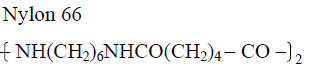B :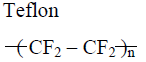C :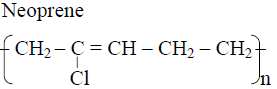D :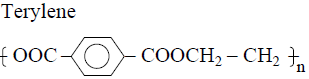•
.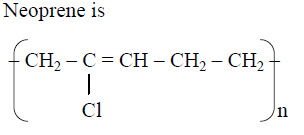•   Question 94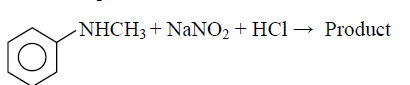A :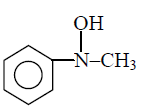B :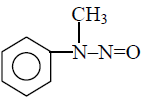C :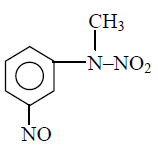D :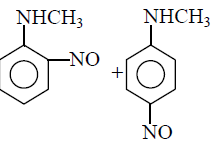•
.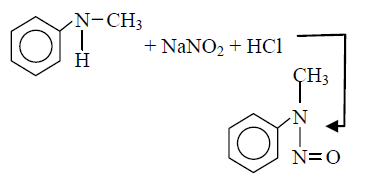•   Question 95

Propionic acid with Br2 / P yields a dibromo product, Its structure would be

A :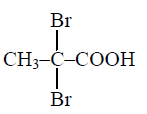B : CH2Br-CHBr-COOH
C :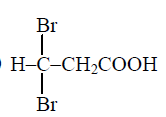D : CH2Br-CH2-COBr

•
.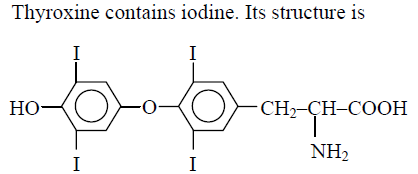•   Question 96

Trichloroacetaldehyde, CCl3CHO reacts with chlorobenzene in presence of sulphuric acid and produces

A :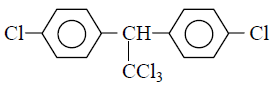B :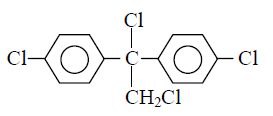C :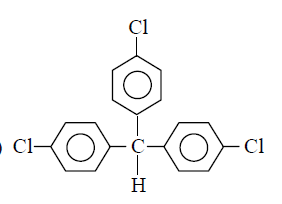D :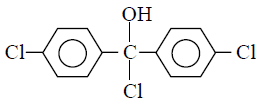•
.•   Question 97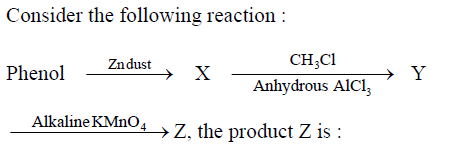A : Benzene
B : Toluene
C : Benzaldehyde
D : Benzoic acid

•
.•   Question 98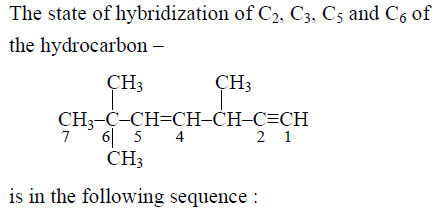A : sp, sp2, sp3 and sp2
B : sp, sp3, sp2 and sp3
C : sp3, sp2, sp2 and sp
D : sp, sp2, sp2 and sp3

•
.•   Question 99

The segment of DNA which acts as the instrument manual for the synthesis of the protein is :

A : Nucleoside
B : Nucleotide
C : Ribose
D : Gene

•
.

•   Question 100

Which of the following hormones contains iodine ?

A : Thyroxine
B : Insuline
C : Testosterone

•
.•   Question 101

Which one of the following has haplontic life cycle ?

A : Wheat
B : Funaria
C : Polytrichum
D : Ustilago

•
.

•   Question 102

T.O.Diener discovered a :

A : Bacteriophage
B : Free infection DNA
C : Free infectious RNA
D : Infectious protein

•
.

•   Question 103

Mannitol is the stored food in -

A : Gracillaria
B : Chara
C : Porphyra
D : Fucus

•
.

•   Question 104

Which one of the following is a vascular cryptogram ?

A : Cedrus
B : Equisetum
C : Ginkgo
D : Marchantia

•
.

•   Question 105

Phylogenetic system of classification is based on:

A : Floral characters
B : Evolutionary relationships
C : Morphological features
D : Chemical constituents

•
.

•   Question 106

Which one of the following groups of animals is bilaterally symmetrical and triploblastic ?

A : Sponges
B : Coelentrates (Cnidarians)
C : Aschelminthes (round worms)
D : Ctenophores

•
.

•   Question 107

Peripatus is a connecting link between :

A : Coelenterata and Porifera
B : Ctenophora and Platyhelminthes
C : Mollusca and Echinodermata
D : Annelida and Arthropoda

•
.

•   Question 108

Which one of the following pairs of animals comprises 'Jawless fishes' ?

A : Guppies and hag fishes
B : Lampreys and eels
C : Mackerals and Rohu
D : Lampreys and hag fishes

•
.

•   Question 109

If a live earthworm is pricked with a needle on its outer surface without damaging its gut, the fluid that comes out is :

A : Slimy mucus
B : excretory fluid
C : Coelomic fluid
D : haemolymph

•
.

•   Question 110

Plasmodesmata are :

A : Connection between adjacent cells
B : Lignified cemented layers between cells
C : Locomotory structures
D : Membranes connecting the nucleus with plasmalemma

•
.

•   Question 111

Stroma in the chloroplast of higher plant contains :

A : Stroma in the chloroplast of higher plant contains :
B : Light-independent reaction enzymes
C : Light-dependent reaction enzymes
D : Ribosomes

•
.

•   Question 112

Synapsis occurs between :

A : two homolog chromosomes
B : a male and a female gamete
C : mRNA and ribosomes
D : spindle fibres and centromere

•
.

•   Question 113

Middle lamella is composed mainly of :

A : Phosphoglycerides
B : Hemicellulose
C : Muramic acid
D : Calcium pectate

•
.

•   Question 114

Cytoskeleton is made up of :

A : Proteinaceous filaments
B : Calcium carbonate granules
C : Callose deposits
D : Cellulose microfibrils

•
.

•   Question 115

The cell junctions called tight, adhering and gap junctions are found in

A : Neural tissue
B : Muscular tissue
C : Connective tissue
D : Epithelial tissue

•
.

•   Question 116

The kind of tissue that forms the supportive structure in our pinna (external ears) is also found in

A : tip of the nose
B : vertebrae
C : nails
D : ear ossicles

•
.

•   Question 117

The epithelial tissue present on the inner surface of bronchioles and fallopian tubes is :

A : Squamous
B : Cuboidal
C : Glandular
D : Ciliated

•
.

•   Question 118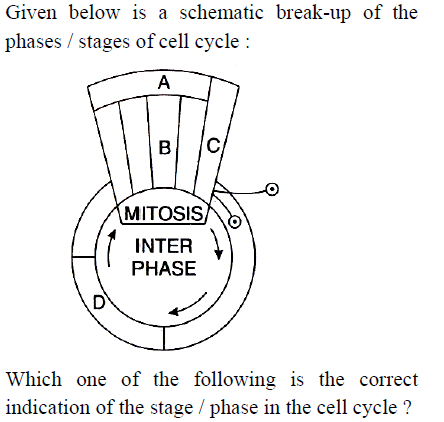A : A-Cytokinesis
B : B-Metaphase
C : C-Karyokinesis
D : D-Synthetic phase

•
.

•   Question 119

What is not true for genetic code?

A : It is unambiguous
B : A codon in mRNA is read in a noncontiguous fashion
C : It is nearly universal
D : It is degenerate

•
.

•   Question 120

Removal of introns and joining the exons in a defined order in a transcription unit is called :

A : Capping
B : Splicing
C : Tailing
D : Transformation

•
.

•   Question 121

Semiconservative replication of DNA was first demonstrated in :

A : Salmonella typhimurium
B : Drosophila melanogaster
C : Escherichia coli
D : Streptococcus pneumoniae

•
.

•   Question 122

Whose experiments cracked the DNA and discovered unequivocally that a genetic code is a "triplet" ?

B : Nirenberg and Mathaei
C : Hershey and Chase
D : Morgan and Sturtevant

•
.

•   Question 123

Point mutation involves :

A : Deletion
B : Insertion
C : Change in single base pair
D : Duplication

•
.

•   Question 124

In the case of peppered moth (Biston betularia) the black-coloured from became dominant over the light-coloured form in England during industrial revolution. This is an example of

A : Inheritance of darker colour character acquired due to the darker environment
B : Natural selection whereby the darker forms were selected.
C : Appearance of the darker coloured individuals due to very poor sunlight
D : Protective mimicry

•
.

•   Question 125

Sickel cell anemia is :

A : Characterized by elongated sickle like RBCs with a nucleus
B : An autosomal linked dominant trait
C : Caused by substitute of valine by glutamic acid in the beta globin chain of haemoglobin
D : Caused by a change in a single base pair of DNA

•
.

•   Question 126

Study the pedigree chart given below
What does it show ?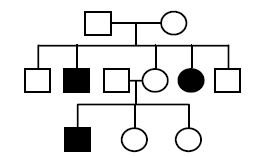A : Inheritance of a recessive sex-linked disease like haemophilia
B : Inheritance of a sex-linked inborn error of metabolism like phenylketonuria
C : Inheritance of a condition like phenylketonuria as an autosomal recessive trait
D : The pedigree chart is wrong as this is not possible

•
.

•   Question 127

The most popularly known blood grouping is the ABO grouping. It is named ABO and not ABC, because "O" in it refers to having :

A : No antigens A and B on RBCs
B : Other antigens besides A and B on RBCs
C : Overdominance of this type on the genes for A and B types
D : One antibody only-either anti-A and anti-B on the RBCs

•
.

•   Question 128

Select the incorrect statement from the following :

A : Baldness is a sex limited trait
B : Linkage is an exception to the principle of independent assortment in heredity.
C : Galactosemia is an inborn error of metabolism
D : Small population size results in random genetic drift in a population

•
.

•   Question 129

Cotyledons and testa respectively are edible parts in

A : Cashew nut and litchi
B : Groundnut and pomegranate
C : Walnut and tamarind
D : French bean and coconut

•
.

•   Question 130

An example of a seed with endosperm, perisperm and caruncle is

A : Castor
B : Cotton
C : Coffee
D : Lily

•
.

•   Question 131

Guard cells help in :

A : Fighting against infection
B : Protection against grazing
C : Transpiration
D : Guttation

•
.

•   Question 132

Manganese is required in

A : Chlorophyll synthesis
B : Nucleic acid synthesis
C : Plant cell wall formation
D : Photolysis of water during photosynthesis

•
.

•   Question 133

Oxygenic photosynthesis occurs in :

A : Chlorobium
B : Chromatium
C : Oscillatoria
D : Rhodospirillum

•
.

•   Question 134

A fruit developed from hypanthodium inflorescence is called :

A : Caryopsis
B : Hesperidium
C : Sorosis
D : Syconus

•
.

•   Question 135

The annular and spirally thickened conducting elements generally develop in the protoxylem when the root or stem is

A : Differentiating
B : Maturing
C : Elongating
D : Widening

•
.

•   Question 136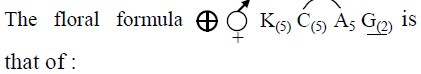A : Tobacco
B : Tulip
C : Soybean
D : Sunnhemp

•
.

•   Question 137

An example of axile placentation is

A : Marigold
B : Argemone
C : Dianthus
D : Lemon

•
.

•   Question 138

In barley stem vascular bundles are

B : Open and scattered
C : Closed and scattered
D : Open and in a ring

•
.

•   Question 139

Aerobic respiratory pathway is appropriately termed

A : Anabolic
B : Catabolic
C : Parabolic
D : Amphibolic

•
.

•   Question 140

Palisade parenchyma is absent in leaves of

A : Gram
B : Sorghum
C : Mustard
D : Soybean

•
.

•   Question 141

Reduction in vascular tissue, mechanical tissue and cuticle is characteristic of :

A : Hydrophytes
B : Xerophytes
C : Mesophytes
D : Epiphytes

•
.

•   Question 142

Anatomically fairly old dicotyledonous root is distinguished from the dicotyledonous stem by :

A : Position of protoxylem
B : absence of secondary xylem
C : Absence of secondary phloem
D : Presence of cortex

•
.

•   Question 143

Cyclic photophosphorylation results in the formation of :

A : ATP
D : ATP, NADPH and O2

•
.

•   Question 144

In a standard ECG which one of the following alphabets is the correct represention of the respective activity of the human heart ?

A : P-depolarisation of the atria
B : R-repolarisation of ventricles
C : S-start of systole
D : T-end of diastole

•
.

•   Question 145

Uric acid is the chief nitrogenous component of the excretory products of

A : Frog
B : Man
C : Earthworm
D : Cockroach

•
.

•   Question 146

Which one of the following pairs of food compounds in humans reaches the stomach totally undigested

A : Starch and cellulose
B : Protein and starch
C : Starch and fat
D : Fat and cellulose

•
.

•   Question 147

Which one of the following is correct pairing of a body part and like kind of muscle tissue that moves it ?

A : Iris � Involuntary smooth muscle
B : Heart wall � Involuntary unstriated muscle
C : Biceps of upper arm � smooth muscle fibres
D : Abdominal wall � smooth muscle

•
.

•   Question 148

Compared to blood our lymph has :

A : More RBCs and less WBCs
B : No plasma
C : Plasma without proteins
D : More WBCs and no RBCs

•
.

•   Question 149

What will happen if the stretch receptors of the urinary bladder wall are totally removed ?

A : There will be no micturition
B : Urine will continue to collect normally in bladder
C : Micturition will continue
D : Urine will not collect in the bladder

•
.

•   Question 150

Which part of human brain is concerned with the regulation of body temperature ?

A : Hypothalamus
B : Medulla Oblongata
C : Cerebellum
D : Cerebrum

•
.

•   Question 151

a young infant may be feeding entirely on mother's milk which is white in colour but the stools which the infant passes out is quite yellowish. What is this yellow colour due to ?

A : Pancreatic juice poured into duodenum
B : Intestinal juice
C : Bile pigments passed through bile juice
D : Undigested milk protein casein

•
.

•   Question 152

Globulins contained in human blood plasma are primarily involved in

A : Clotting of blood
B : Defence mechanisms of body
C : Osmotic balance of body fluids
D : Oxygen transport in the blood

•
.

•   Question 153

Seminal plasma in humans is rich in :

A : Fructose and certain enzymes but poor calcium
B : Fructose and calcium but has no enzyme
C : Fructose, calcium and certain enzymes
D : Glucose and certain enzymes but has no calcium

•
.

•   Question 154

Given below is a diagrammatic sketch for a portion of human male reproductive system. Select the correct set of the names of the parts labelled A, B, C, D respectively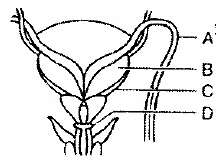A : Ureter Seminal, vesicle, Prostate, Bulbourethral gland
B : Ureter ,Prostate ,Seminal vesicle ,Bulbouretharal gland
C : Vas deferens ,Seminal vesicle ,Prostate ,Bulbourethral gland
D : Vas deferens ,Seminal vesicle ,Bulbourethral gland ,Prostate

•
.

•   Question 155

Which one of the following is the correct matching of three items and their grouping category ?
Items=Groups

A : Cytosine, uracil, thiamine= Pyrimidines
B : Malleus, incus, cochlea =Ear ossicles
C : ilium, ischium pubis =Coxal bones of pelvic girdle
D : Actin, myosin, rodopsin =Muscle proteins

•
.

•   Question 156

Which one of the following statement is true regarding digestion and absorption of food in humans

A : About 60% of starch is hydrolysed by salivary amylase in our mouth
B : Oxyntic cells in our stomach secrete the proenzyme pepsinogen
C : Fructose and amino acids are absorbed through intestinal mucosa with the help of carrier ions like Na+
D : Chylomicrons are small lipoprotein particles that are transported from intestine into blood capillaries

•
.

•   Question 157

Which one of the following correctly described the location of some body parts in the earthworm Pheretima ?

A : Two pairs of accessory glands in 16�18 segments
B : Two pairs of testes in 10th and 11th segments
C : Four pairs of spermathecae in 4�7 segments
D : One pair of ovaries attached at intersegmental septum of 14th and 15 th segments.

•
.

•   Question 158

Elbow joint is an example of :

A : Ball and socket joint
B : Pivot joint
C : Hinge joint
D : Gliding joint

•
.

•   Question 159

Which one of the following is considered important in the development of seed habit ?

A : Free-living gametophyte
B : Dependent sporophyte
C : Heterospory
D : Haplontic life cycle

•
.

•   Question 160

One of the synthetic auxin is :

A : IBA
B : NAA
C : IAA
D : GA

•
.

•   Question 161

Which one of the following acids is a derivative of carotenoids ?

A : Abscisic acid
B : Indole butyric acid
C : Indole-3-acetic acid
D : Gibberellic acid

•
.

•   Question 162

Vegetative propagation in mint occurs by :

A : Sucker
B : Runner
C : Offset
D : Rhizome

•
.

•   Question 163

Which one of the following plants is monoecios ?

A : Papaya
B : Marchantia
C : Pinus
D : Cycas

•
.

•   Question 164

Foetal ejection reflex in human female is induced by

A : Differentiation of mammary glands
B : Pressure exerted by amniotic fluid
C : Release of oxytocin from pituitary
D : Fully developed foetus and placenta

•
.

•   Question 165

Which of the following is the correct matching of the events occurring during menstrual cycle ?

A : Menstruation- Breakdown of myometrium and ovum not fertilized
B : Ovulation- LH and FSH attain peak level and sharp fall in the secretion of progesterone
C : Proliferative phase- Rapid regeneration of myometrium and maturation of Grafian follicle
D : Development of corpus luteum- Secretory phase and increased secretion of progesterone.

•
.

•   Question 166

Which one of the following is the most likely root cause why menstruation is not taking place in regularly cycling human female ?

A : Retention of well-developed corpus luteum
B : Fertilization of the ovum
C : Maintenance of the hypertrophical endometrial lining
D : Maintenance of high concentration of sexhormones in the blood stream

•
.

•   Question 167

The correct sequence of spermatogenetic stages leading to the formation of sperms in a mature human testis is :

A : Spermatogonia-spermatid-spermatocytesperms
B : Spermatocyte-spermatogonia-spermatidsperms
C : Spermatogonia-spermatocyte-spermatidsperms
D : Spermatid-spermatocyte-spermatogoniasperms

•
.

•   Question 168

A change in the amount of yolk and its distribution in the egg will effect

A : Fertilization
B : Formation of zygote
C : Pattern of cleavage
D : Number of blastomeres produced

•
.

•   Question 169

When breast feeding is replaced by less nutritive food low in proteins and calories, the infants below the age of one year are likely to suffer from :

A : Pellagra
B : Marasmus
C : Rickets
D : Kwashiorkor

•
.

•   Question 170

Which one of the following types organisms occupy more than one trophic level in a pond ecosystem ?

A : Frog
B : Phytoplankton
C : Fish
D : Zooplankton

•
.

•   Question 171

Which one of the following has maximum genetic diversity in India ?

A : Tea
B : Teak
C : Mango
D : Wheat

•
.

•   Question 172

Montreal protocol aims at :

A : Control of CO2 emission
B : Reduction of ozone depleting substances
C : Biodiversity conservation
D : Control of water pollution

•
.

•   Question 173

Chipko movement was launched for the protection of

A : Wet lands
B : Grasslands
C : Forests
D : Livestock

•
.

•   Question 174

The correct sequence of plants in hydrosere is

A : Oak - Lantana - Volvox - Hydrilla - Pistia - Scirpus
B : Oak - Lantana - Scirpus - Pistia - Hydrilla - Volvox
C : Volvox - Hydrilla - Pistia - Scirpus - Lantana - Oak
D : Pistia - Volvox - Scirpus - Hydrilla - Oak - Lantana

•
.

•   Question 175

A country with a high rate of population growth took measures to reduce it. The figure below shows age-sex pyramids of populations A and B twenty years apart. Select the correct interpretation about them :A : "A" is the earlier pyramid and no change has occurred in the growth rate
B : "A" is more recent shows slight reduction in the growth rate
C : "B" is earlier pyramid and shows stabilized growth rate
D : "B" is more recent showing that population is very young

•
.